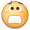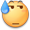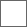### 17-04-06【资料数据】MHXX近战紫斩补正变更测试汇总

MH4G中斩味补正为

MHX删除了紫斩，但是延续了4G其他斩味的补正，而MHXX紫斩回归后大幅更改了紫斩的物理补正。
MHXX斩味补正为：

（动作值 × 斩味补正 × 会心补正 × 攻击力 × 肉质）+ （属性值 × 斩味补正 × 属性会心补正 × 肉质）=粗略伤害

3级螳螂斩斧330 紫 无会心

32 × 1.2 =38.4切捨=38（实际动作值）
38 × 1× 3.3 × 0.9=112.86切捨=112
112 × 0.8 =89.6切捨=89 （黄斩）
38 × 1.05× 3.3 × 0.9 切捨=118
118 × 0.8 切捨=94（绿斩）
38 × 1.2× 3.3 × 0.9 切捨=135
135 × 0.8 =108（蓝斩）
38 × 1.32× 3.3 × 0.9 切捨=148
148 × 0.8 切捨=118（白斩）
38 × 1.45× 3.3 × 0.9 切捨=163
163 × 0.8 切捨=130（原紫斩）

38 × 1.37 × 3.3 × 0.9 切捨=154
154 × 0.8 切捨
38 × 1.38 × 3.3 × 0.9 切捨=155
155 × 0.8 切捨
38 × 1.39 × 3.3 × 0.9 切捨=156
156 × 0.8 切捨
38 × 1.4 × 3.3 × 0.9 切捨=158
158 × 0.8 切捨

3级螳螂斩斧330 紫 无会心

38 × 1.38 × 3.3 × 0.56 切捨=96
38 × 1.39 × 3.3 × 0.56 切捨=97
38 × 1.38 × 3.3 × 0.6 切捨=103
38 × 1.39 × 3.3 × 0.6 切捨=104
38 × 1.38 × 3.3 × 0.65 切捨=112
38 × 1.39 × 3.3 × 0.65 切捨=113
48 × 1.38 × 3.6 × 0.9 切捨=214
48 × 1.39 × 3.6 × 0.9 切捨=216

3级祖龙大剑300攻 75龙 紫

48 × 1.39 × 3.0 × 0.9 = 180.144
75 × 1.2 × 0.35 + 180.144 = 211.644
75 × 1.21 × 0.35 + 180.144 = 211.9065

48 × 1.39 × 3 × 0.65 = 130.104
75 × 1.19 × 0.3 + 130.104 =156.879
75 × 1.2 × 0.3 + 130.104 =157.104
75 × 1.23 × 0.3 + 130.104 =157.779
75 × 1.24 × 0.3 + 130.104 =158.004

48 × 1.39 × 3 × 0.2 = 40.032
75 × 1.2 × 0.3 + 40.032 = 67.032
75 × 1.24 × 0.3 + 40.032 =67.9320.12*1.39*0.8*266…35.49

327*0.12*0.8*1.32…41.43

327*0.12*0.8*1.39…43.63MHW怪猎世界加速器用迅游,解决登入困难,组队失败,加入集会所困难,联机卡顿新用户免费体验 低延迟 0丢包 超稳定

2019-03-21 16:12 广告347以上白斩期望高于330紫斩
315以上白斩挑2期望高于330紫斩如果真是这样的话，那就不用纠结于紫斩了。
不过，我总觉得X系列的制作人，一直在削弱以前作品中的某些东西，匠的15点，武士道直接让回性和耳栓变的可有可无。虫棍吸个白就能当心眼用，等等。

然而斩斧依然是螳螂独大，倍率比螳螂高的要么要填负会，要么匠2才有长白，还不如继续匠二长紫螳螂刚才拿岩龙测了一下，如果岩龙背肉质还是15，上位弹刀值还是25的话
大剑紫斩二蓄 如果紫斩补正1.38,15*1.2*1.38=24.84弹刀
如果是1.39,15*1.2*1.39=25.02不弹刀
实测不弹刀，那应该是1.39借帖问下超会心有没有变？

收起回复惊了，我就知道天慧武器这样弄是有预谋的

反正天彗武器是没之前那么废物了

？？？补丁改补正螳螂武器的史诗级削弱？如果是1.1补丁导致的，我觉得有可能是卡婊数值改错了看了一下，大剑仍然是狗龙，斩斧仍然是螳螂，盾斧仍然是角王，太刀铠裂能和岩穿争一下官方打压近战必匠的手段

#### 扫二维码下载贴吧客户端

下载贴吧APP
看高清直播、视频！